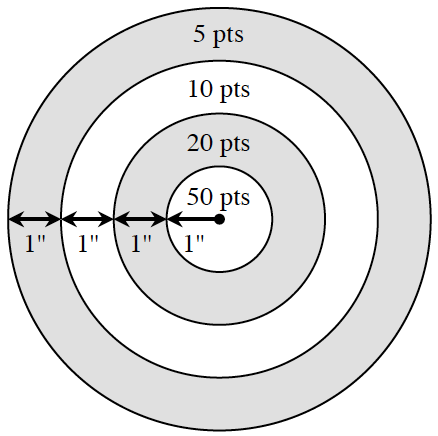### Home > INT3 > Chapter 8 > Lesson 8.3.3 > Problem8-136

8-136.

In one of the games at the County Fair, people pay to shoot a paint pistol at the target shown at right. The center has a radius of one inch. Each concentric circle has a radius one inch larger than the preceding circle. Assuming the paint pellet hits the target randomly, what is the probability it hits:

1. The $50$ point ring?

What is the area of the $50$ point ring?
What is the area of the whole target?

2. The $20$ point ring?

Calculate the area of the circle around the $20$ point ring.
Then subtract the area of the $50$ point ring.

$\frac{3}{16}$